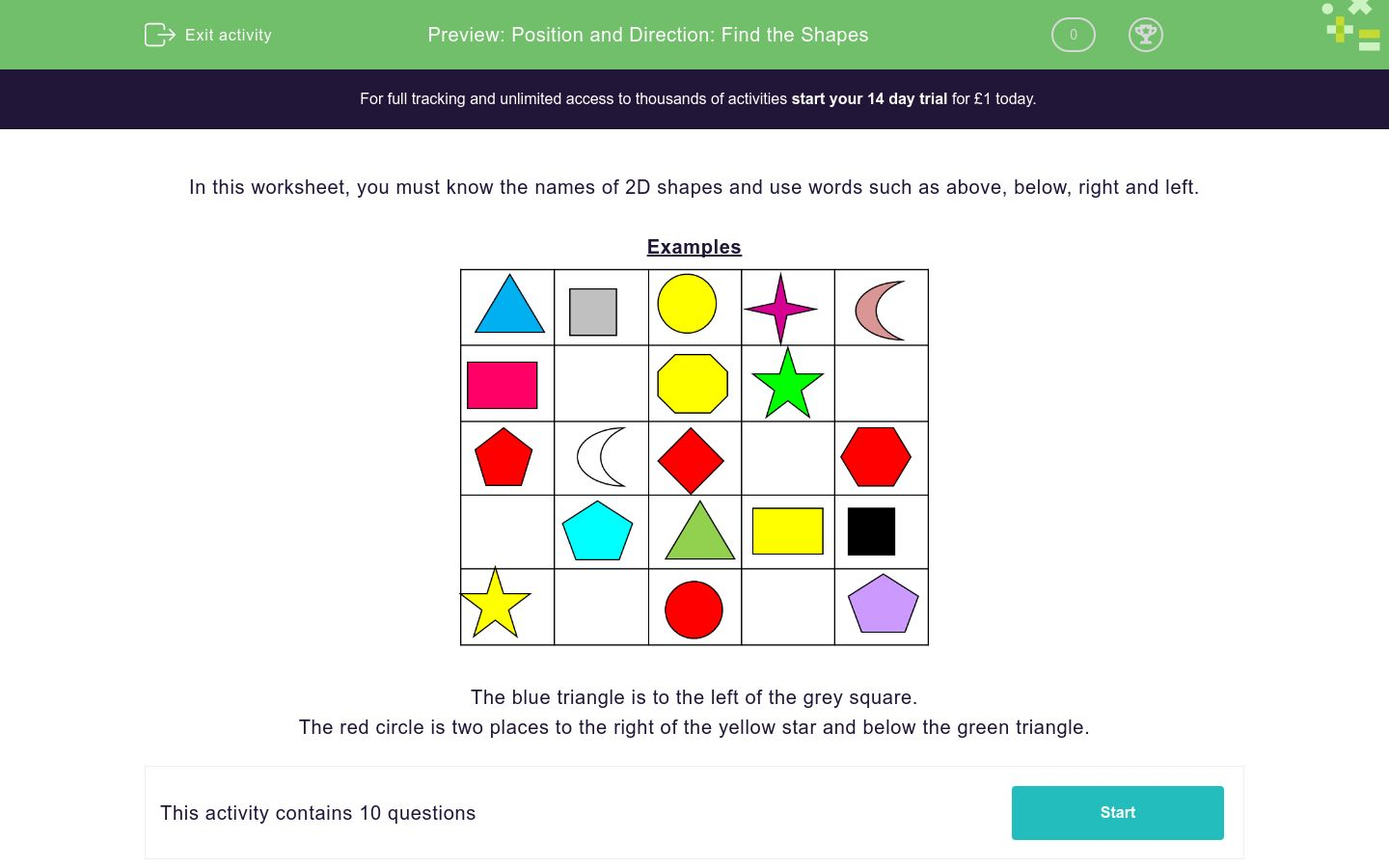# Position and Direction: Find the Shapes

In this worksheet, students identify 2D shapes and understand words relating to their position in a grid.Key stage:  KS 1

Curriculum topic:   Geometry: Position and Direction

Curriculum subtopic:   Use Language of Position/Direction

Difficulty level:### QUESTION 1 of 10

In this worksheet, you must know the names of 2D shapes and use words such as above, below, right and left.

ExamplesThe blue triangle is to the left of the grey square.

The red circle is two places to the right of the yellow star and below the green triangle.

Look at this grid.

Which shape is to the left of the yellow circle?Purple star

Grey square

Red square

Look at this grid.

Which shape is to the left of the red circle?Nothing

Green Triangle

Purple Pentagon

Look at this grid.

Tick all the shapes which are next to the black square?Red pentagon

Red hexagon

Yellow rectangle

Purple pentagon

Look at this grid.

Tick all the shapes which are next to the grey square?Rectangle

Triangle

Circle

Pentagon

Look at this grid.

Which shape is below the yellow circle?Pentagon

Octagon

Hexagon

Look at this grid.

Which shape is below the red square? (search hard for it.....it may not be upright!)Pentagon

Circle

Triangle

Look at this grid.

Which shape is two places to the right of the blue triangle?Square

Circle

Triangle

Look at this grid.

Which shape is two places above the red circle?Square

Circle

Triangle

Look at this grid.

Which shape is four places below the yellow circle?Square

Circle

Triangle

Look at this grid.

Which shape is three places to the left of the black square?Rectangle

Pentagon

Triangle

• Question 1

Look at this grid.

Which shape is to the left of the yellow circle?Grey square
• Question 2

Look at this grid.

Which shape is to the left of the red circle?Nothing
• Question 3

Look at this grid.

Tick all the shapes which are next to the black square?Red hexagon
Yellow rectangle
Purple pentagon
• Question 4

Look at this grid.

Tick all the shapes which are next to the grey square?Triangle
Circle
• Question 5

Look at this grid.

Which shape is below the yellow circle?Octagon
• Question 6

Look at this grid.

Which shape is below the red square? (search hard for it.....it may not be upright!)Triangle
• Question 7

Look at this grid.

Which shape is two places to the right of the blue triangle?Circle
• Question 8

Look at this grid.

Which shape is two places above the red circle?Square
• Question 9

Look at this grid.

Which shape is four places below the yellow circle?Circle
• Question 10

Look at this grid.

Which shape is three places to the left of the black square?Pentagon
---- OR ----

Sign up for a £1 trial so you can track and measure your child's progress on this activity.

### What is EdPlace?

We're your National Curriculum aligned online education content provider helping each child succeed in English, maths and science from year 1 to GCSE. With an EdPlace account you’ll be able to track and measure progress, helping each child achieve their best. We build confidence and attainment by personalising each child’s learning at a level that suits them.

Get started